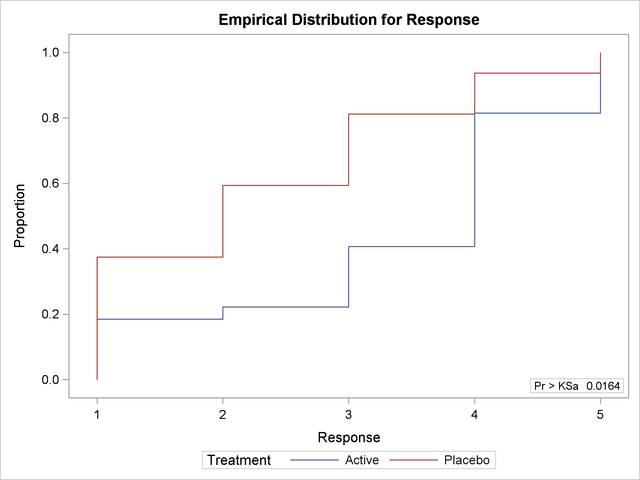## Example 64.2 EDF Statistics and EDF Plot

This example uses the SAS data set Arthritis created in Example 64.1. The data set contains the variable Treatment, which denotes the treatment received by a patient, and the variable Response, which contains the response status of the patient. The variable Freq contains the frequency of the observation, which is the number of patients with the Treatment and Response combination.

The following statements request empirical distribution function (EDF) statistics, which test whether the distribution of a variable is the same across different groups. The EDF option requests the EDF analysis. The variable Treatment is the CLASS variable, and the variable Response specified in the VAR statement is the analysis variable. The FREQ statement names Freq as the frequency variable.

The PLOTS= option requests an EDF plot for Response classified by Treatment. ODS Graphics must be enabled before producing plots.

```ods graphics on;
proc npar1way edf plots=edfplot data=Arthritis;
class Treatment;
var Response;
freq Freq;
run;
ods graphics off;
```

Output 64.2.1 shows EDF statistics that compare the two levels of Treatment, Active and Placebo. The asymptotic p-value for the Kolmogorov-Smirnov test is 0.0164. This supports rejection of the null hypothesis that the distributions are the same for the two samples.

Output 64.2.2 shows the EDF plot for Response classified by Treatment.

Output 64.2.1 Empirical Distribution Function Statistics
The NPAR1WAY Procedure

Kolmogorov-Smirnov Test for Variable Response
Classified by Variable Treatment
Treatment N EDF at
Maximum
Deviation from Mean
at Maximum
Active 27 0.407407 -1.141653
Placebo 32 0.812500 1.048675
Total 59 0.627119

Kolmogorov-Smirnov Two-Sample Test
(Asymptotic)
KS 0.201818 D 0.405093
KSa 1.550191 Pr > KSa 0.0164

Output 64.2.2 Empirical Distribution Function Plot